# Using the IF AND OR Functions in Microsoft Excel

In this article, we will learn to apply multiple conditions in a single formula using OR and AND function with the IF function.

IF function in Excel is used to check the condition and returns value on the basis of it.
Syntax:

=IF(Logic_test, [Value_if True], [Value_if_False])

OR function works on logic_test. It helps you run multiple conditions in Excel. If any one of them is True, OR function returns True else False.
Syntax:

=OR( Logic_test 1, [logic_test 2], ..)

AND function works on logic_test. It helps you run multiple conditions in Excel. If every one of them is True, then only AND function returns True else False.
Syntax:

=AND( Logic_test 1, [logic_test 2], ..)

First we will use IF with OR function.

Let’s get this by an example here.
We have a list of months and need to know in which quarter it lay.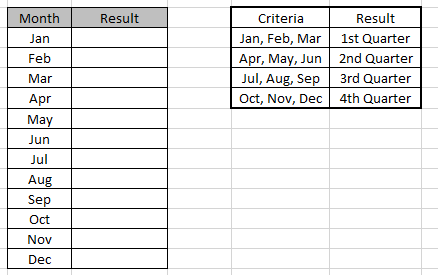Use the formula to match the quarter of the months

Formula:

=IF(OR(A4=”Jan”,A4=”Feb”,A4=”Mar”),”1st
Quarter”,IF(OR(A4=”Apr”,A4=”May”,A4=”Jun”),”2nd
Quarter”,IF(OR(A4=”Jul”,A4=”Aug”,A4=”Sep”),”3rd
Quarter”,IF(OR(A4=”Oct”,A4=”Nov”,A4=”Dec”),”4th Quarter”))))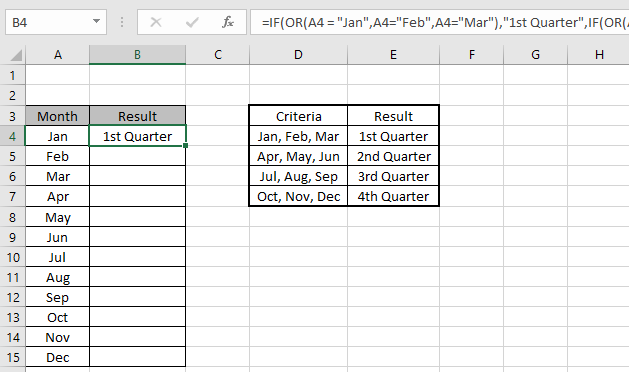Copy the formula in other cells, select the cells taking the first cell where the formula is already applied, use shortcut key Ctrl +D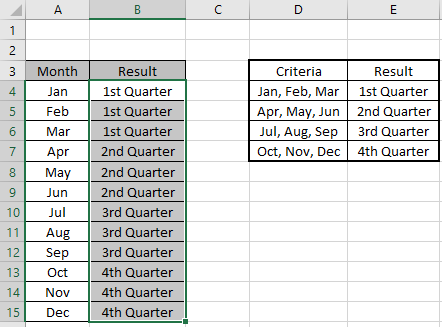We got the result.
You can use IF and OR function to meet multiple conditions in a single formula.

Now we will use IF with AND function in Excel.

Let’s get this by an example here.
We have a list of Scores and We need to know under which criteria it lay.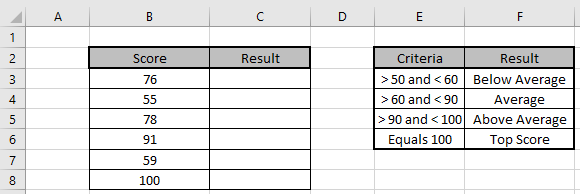Use the formula to match the criteria

Formula:

=IF(AND(B3 > 50, B3 < 60), "Below Average",IF(AND(B3 > 60, B3 < 90),"Average",IF(AND(B3 > 90,B3< 100),"Above Average","Top Score")))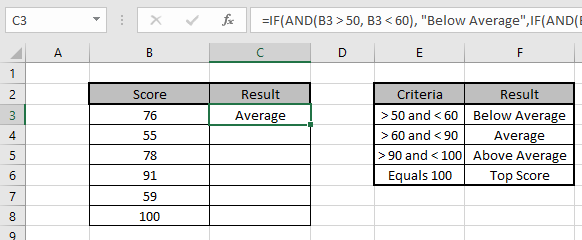Copy the formula in other cells, select the cells taking the first cell where the formula is already applied, use shortcut key Ctrl + D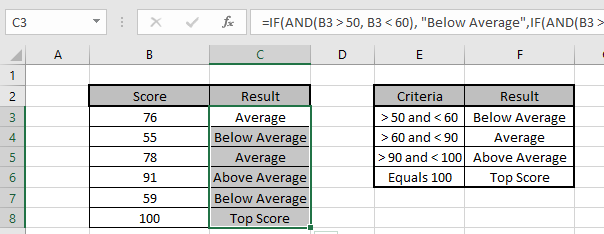We got the Results corresponding to the Scores.
You can use IF and AND function to meet multiple conditions in a single formula.

Hope you understood IF, AND and OR function in Excel 2016. Find more articles on Logic_test here. Please share your query below in the comment box. We will assist you.

1.